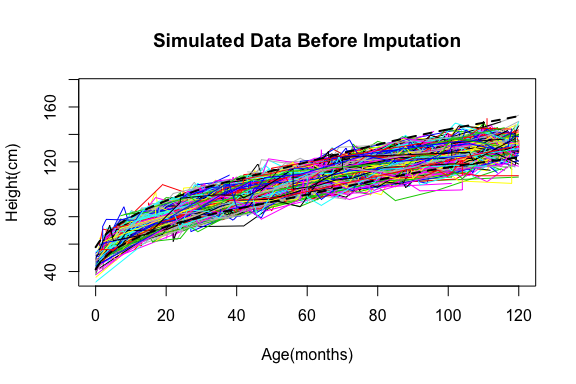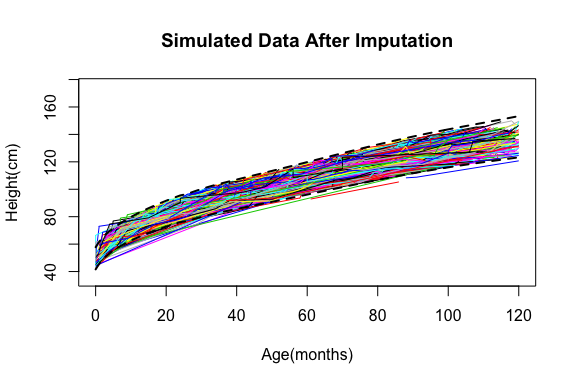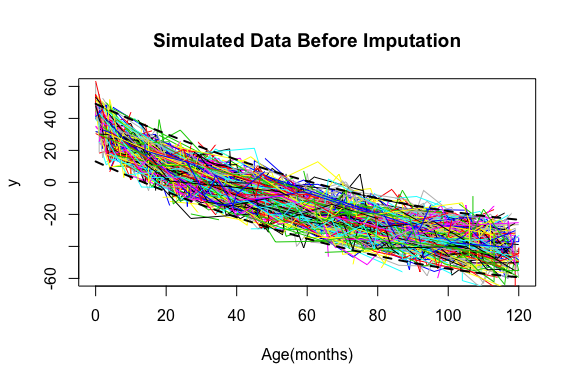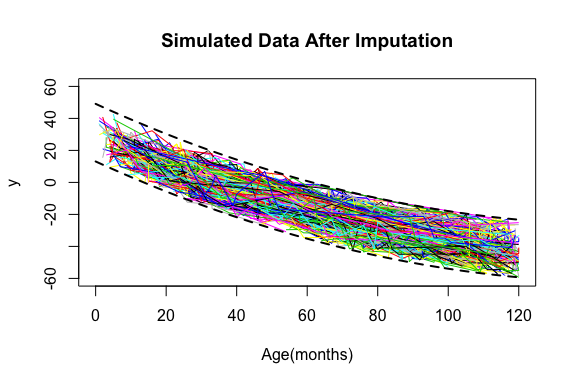# MonoInc R Package

The MonoInc package in R seeks to clean data so that erroneous values are less effective. Given a prespecified range, MonoInc will determine if an observation is “unusual”, then replace the value if so desired. MonoInc will impute on participant data individually, so the number of time points need not be the same.

###### simulated_data

This data was simulated to imitate height growth of female children in electronic medical records. There are 500 individuals, with a random number of data points. Based on the CDC growth curve, each individual has a two-level random effect (intercept and slope), a common intercept, and a random error term. The ages range from 0 to 10 years, which is given in whole months.}

head(simulated_data, n=20)
   nestid age    height
1       1  13  66.94740
2       1  27  82.41612
3       1  42  98.21975
4       1  44  95.80379
5       1  46 100.33827
6       1  58 106.61578
7       1  86 108.70443
8       1 117 132.88932
9       2  27  88.04768
10      2  33 101.04429
11      2  66 101.17805
12      2  73 115.26163
13      2 102 136.64803
14      3  13  72.80796
15      3  36  97.88857
16      3  52 105.81838
17      3  53  95.88865
18      3  65 114.83026
19      3  66 107.66502
20      3 103 129.23952
###### data.r

CDC growth chart of heights of female children aged 0 to 120 months.

head(data.r, n=10)
   Age    Per_5   Per_95
1    0 45.57561 53.77291
2    1 49.72160 57.67502
3    2 52.82952 60.77038
4    3 55.30621 63.34020
5    4 57.41683 65.58764
6    5 59.28178 67.61052
7    6 60.96766 69.46519
8    7 62.51583 71.18770
9    8 63.95401 72.80276
10   9 65.30182 74.32825
###### simDEC_data

This data was simulated to be monotone decreasing. There are 500 individuals, with a random number of data points. Each individual has a two-level random effect (intercept and slope), a com- mon intercept, and a random error term. The ages range from 0 to 10 years, which is given in whole months.

head(simDEC_data, n=20)
   id age          y
1   1  12  27.132657
2   1  12  17.309024
3   1  23   8.019683
4   1  45  -8.031288
5   1  64 -17.037672
6   1  71         NA
7   1  74 -16.933872
8   1  76 -26.270909
9   1  78 -24.392502
10  1  95 -40.729580
11  1 100 -38.352936
12  1 100 -29.675448
13  1 111 -40.275939
14  1 114 -37.240150
15  1 118 -43.210878
16  2  68 -10.445063
17  2  94         NA
18  3  33         NA
19  3  71         NA
20  4   9         NA
###### decdata.r

Chart of measurements of children aged 0 to 120 months

head(decData.r, n=10)
   Age   L.bound  U.bound
1    0 16.085781 46.08578
2    1 15.083704 45.08370
3    2 14.088330 44.08833
4    3 13.099658 43.09966
5    4 12.117688 42.11769
6    5 11.142421 41.14242
7    6 10.173856 40.17386
8    7  9.211994 39.21199
9    8  8.256833 38.25683
10   9  7.308376 37.30838

## mono.flag

This function flags the data that is outside a prespeficied range(data.r) and that is not monotone increasing.

Usage

mono.flag(data, id.col, x.col, y.col, min, max, data.r = NULL, tol = 0, direction)
Arguments
data a data.frame or matrix or vector of measurement data
id.col column where the id’s are stored
x.col column where x values, or time variable is stored
y.col column where y values, or measurements are stored
min lowest acceptable value for measurement; does not have to be a number in ycol
max highest acceptable value for measurement; does not have to be a number in ycol
data.r prespecified range for y values; must have three columns: 1 - must match values in xcol, 2 - lower range values, 3 - upper range values
tol tolerance; how much outside of the range (data.r) is acceptable; same units as data in ycol
direction the direction of the function a choice between increasing ‘inc’, and decreasing ‘dec’

Example

test <- mono.flag(simulated_data, 1, 2, 3, 30, 175, data.r=data.r, direction='inc')
Warning: executing %dopar% sequentially: no parallel backend registered
head(test)
  ID  X         Y Decreasing Outside.Range
1  1 13  66.94740      FALSE          TRUE
2  1 27  82.41612      FALSE         FALSE
3  1 42  98.21975      FALSE         FALSE
4  1 44  95.80379       TRUE         FALSE
5  1 46 100.33827      FALSE         FALSE
6  1 58 106.61578      FALSE         FALSE

## mono.range

This function checks how many entries fall inside and outside of the prespecified range.

Usage

mono.range(data, data.r, id.col, tol, xr.col, x.col, y.col)
Arguments
data a data.frame or matrix or vector of measurement data
data.r range for y values; must have three columns: 1 - must match values in x.col, 2 - lower range values, 3 - upper range values
tol tolerance; how much outside of the range (data.r) is acceptable; same units as data in y.col
xr.col column where x values, or time variable is stored in data.r
x.col column where x values, or time variable is stored in data
y.col column where y values, or measurements are stored in data

Example

mono.range(simulated_data, data.r, tol=4, xr.col=1 ,x.col=2, y.col=3)
##  0.6774194

## monotonic

This function can check the monoticity of a single vector or a matrix or data.frame that has multiple IDs with in the matrix or data.frame.

Usage

monotonic(data, id.col=NULL, y.col=NULL, direction)
Arguments
data a data.frame or matrix or vector of measurement data
id.col column where the id’s are stored; default is NULL
y.col column where y values, or measurements are stored; default is NULL
direction the direction of the function a choice between increasing ‘inc’, and decreasing ‘dec’

Examples

#Checking if a vector is Monotonic
x<-c(1,2,3,4,5,6,7)
monotonic(x, direction='inc')
##  TRUE
x<-c(5,4,3,2,1)
monotonic(x,direction='dec')
##  TRUE
x<-c(1,2,7,4,2,6, direction='inc')
monotonic(x)
##  FALSE
#Checking monoticity with mising values
x<-c(1,3,4,5,NA)
monotonic(x, direction='inc') #if there is an NA present, the function will return NA
##  NA
monotonic(na.omit(x), direction='inc') #using na.omit will ignore the NAs 
##  TRUE
#check of a data.matrix has monotonic  data
test <- monotonic(simulated_data, 1,3, direction = 'inc')
head(test)
##      id Montonic
## [1,]  1        0
## [2,]  2        1
## [3,]  3        0
## [4,]  4        0
## [5,]  5        0
## [6,]  6        0
table(as.logical(test[,2])) #look at the number of ids that are non-monotonic
##
## FALSE  TRUE
##   422    78
test <- monotonic(na.omit(simDEC_data), 1,3, direction = 'dec')
head(test)
##      id Montonic
## [1,]  1        0
## [2,]  2        1
## [3,]  4        0
## [4,]  6        0
## [5,]  7        0
## [6,]  8        1
table(as.logical(test[,2])) #look at the number of ids that are non-monotonic
##
## FALSE  TRUE
##   375   103

## MonoInc

Combines many of the functions in the MonoInc package. Given a data range, weights, and imputation methods of choice, MonoInc will impute flagged values using one or a combination of two imputation methods. It can also perform all single imputation methods for comparison.

Usage

MonoInc(data, id.col, x.col, y.col, data.r = NULL, tol = 0, direction = 'inc', w1 = 0.5, min, max, impType1 = 'nn', impType2 = 'reg', sum = FALSE
Arguments
data a data.frame or matrix or vector of measurement data
id.col column where the id’s are stored
x.col column where x values, or time variable is stored
y.col column where y values, or measurements are stored
data.r prespecified range for y values; must have three columns: 1 - must match values in xcol, 2 - lower range values, 3 - upper range values
tol tolerance; how much outside of the range (data.r) is acceptable; same units as data in ycol
direction the direction of the function a choice between increasing ‘inc’, and decreasing ‘dec’
w1 weight of imputation type 1 (impType1); default is 0.50
min lowest acceptable value for measurement; does not have to be a number in ycol
max highest acceptable value for measurement; does not have to be a number in ycol
impType1 imputation method 1, a choice between Nearest Neighbor ‘nn’, Regression ‘reg’, Fractional Regression ‘fr’, Last Observation Carried Forward ‘locf’, or Last & Next ‘ln’; default is ‘nn’
impType2 mputation method 2; default is ‘reg’
sum if true the function will return a matrix of all imputation methods in the package

Examples

# If sum=TRUE, it will return a column for each imputation method
sum <- MonoInc(simulated_data, 1,2,3, data.r,4,direction = 'inc', w1=0.3, min=30, max=175, impType1=NULL, impType2=NULL, sum=T)
head(sum)
##   ID   X         Y Nearest.Neighbor Regression      LOCF Last.Next Fractional.Reg Decreasing Outside.Range
## 1  1  13  66.94740         66.94740   66.94740  66.94740  66.94740       66.94740      FALSE         FALSE
## 2  1  27  82.41612         82.41612   82.41612  82.41612  82.41612       82.41612      FALSE         FALSE
## 3  1  42  98.21975         98.21975   98.21975  98.21975  98.21975       98.21975      FALSE         FALSE
## 5  1  46 100.33827        100.33827  100.33827 100.33827 100.33827      100.33827      FALSE         FALSE
## 6  1  58 106.61578        106.61578  106.61578 106.61578 106.61578      106.61578      FALSE         FALSE
## 8  1 117 132.88932        132.88932  132.88932 132.88932 132.88932      132.88932      FALSE         FALSE
locf <- MonoInc(simulated_data, 1,2,3, data.r,4,direction = 'inc', w1=0.3, min=30, max=175, impType1='locf', impType2=NULL)
head(locf) 
##   ID  X         Y Decreasing Outside.Range
## 1  1 13  66.94740      FALSE         FALSE
## 2  1 27  82.41612      FALSE         FALSE
## 3  1 42  98.21975      FALSE         FALSE
## 4  1 44  98.21975       TRUE         FALSE
## 5  1 46 100.33827      FALSE         FALSE
## 6  1 58 106.61578      FALSE         FALSE
#If two imputation methods are used, MonoInc will take a weighted average of the output of the imputed values
test <- MonoInc(simulated_data, 1,2,3, data.r,4,direction = 'inc', w1=0.3, min=30, max=175, impType1='ln', impType2='reg')
head(test)
##   ID  X    Reg.Ln Decreasing Outside.Range
## 1  1 13  66.94740      FALSE         FALSE
## 2  1 27  82.41612      FALSE         FALSE
## 3  1 42  98.21975      FALSE         FALSE
## 4  1 44  95.59701       TRUE         FALSE
## 5  1 46 100.33827      FALSE         FALSE
## 6  1 58 106.61578      FALSE         FALSEFigure 1: Before Imputation shows the plot of each individuals growth from raw simulated_data. The dotted lines are boundary lines that represent the upper and lower limits from the prespecified data (data.r) with a tolerance of 4.Figure 2: After Imputation shows the plot of each individuals growth from simulated_data after a weighted imputation combining last and next imputation and regression imputation. The dotted lines are boundary lines that represent the upper and lower limits from the prespecified data (data.r) with a tolerance of 4.

sum <- MonoInc(simDEC_data, 1,2,3, decData.r,3,direction = 'dec', w1=0.3, min=-60, max=100, impType1=NULL, impType2=NULL, sum=T)
head(sum)
##    ID  X          Y Nearest.Neighbor Regression       LOCF  Last.Next Fractional.Reg Decreasing Outside.Range
## NA NA NA         NA               NA         NA         NA         NA             NA         NA            NA
## 3   1 23   8.019683         8.019683   8.019683   8.019683   8.019683       8.019683       TRUE         FALSE
## 4   1 45  -8.031288        -8.031288  -8.031288  -8.031288  -8.031288      -8.031288       TRUE         FALSE
## 7   1 74         NA       -26.270909 -21.898960 -17.037672 -21.654290     -20.687254      FALSE         FALSE
## 8   1 76 -26.270909       -26.270909 -26.270909 -26.270909 -26.270909     -26.270909       TRUE         FALSE
## 9   1 78 -24.392502       -24.392502 -24.392502 -24.392502 -24.392502     -24.392502       TRUE         FALSE
locf <- MonoInc(simDEC_data, 1,2,3, decData.r,3,direction = 'dec', w1=0.3, min=-60, max=100, impType1='locf', impType2=NULL)
head(locf) 
##   ID  X          Y Decreasing Outside.Range
## 2  1 12         NA      FALSE         FALSE
## 3  1 23   8.019683       TRUE         FALSE
## 4  1 45  -8.031288       TRUE         FALSE
## 5  1 64 -17.037672       TRUE         FALSE
## 6  1 71 -17.037672      FALSE          TRUE
## 7  1 74 -17.037672      FALSE         FALSE
test <- MonoInc(simDEC_data, 1,2,3, decData.r,3,direction = 'dec', w1=0.3, min=-60, max=100, impType1='ln', impType2='reg')
head(test)
##   ID  X     Reg.Ln Decreasing Outside.Range
## 2  1 12         NA      FALSE         FALSE
## 3  1 23   8.019683       TRUE         FALSE
## 4  1 45  -8.031288       TRUE         FALSE
## 5  1 64 -17.037672       TRUE         FALSE
## 6  1 71 -20.750568      FALSE          TRUE
## 7  1 74 -21.825559      FALSE         FALSEFigure 3: Before Imputation shows the plot of each individuals growth from raw simDEC_data. The dotted lines are boundary lines that represent the upper and lower limits from the prespecified data (decdata.r) with a tolerance of 3Figure 4: After Imputation shows the plot of each individuals growth from simDEC_data after a weighted imputation combining last and next imputation and regression imputation. The dotted lines are boundary lines that represent the upper and lower limits from the prespecified data (decdata.r) with a tolerance of 3

#Please note that you can not compute sum and imputation at te same time
test<-MonoInc(simulated_data, 1,2,3, data.r,4, w1=0.3, min=30, max=175, impType1='ln', impType2='reg', sum=TRUE)
## Error: choose either sum or an imputation method